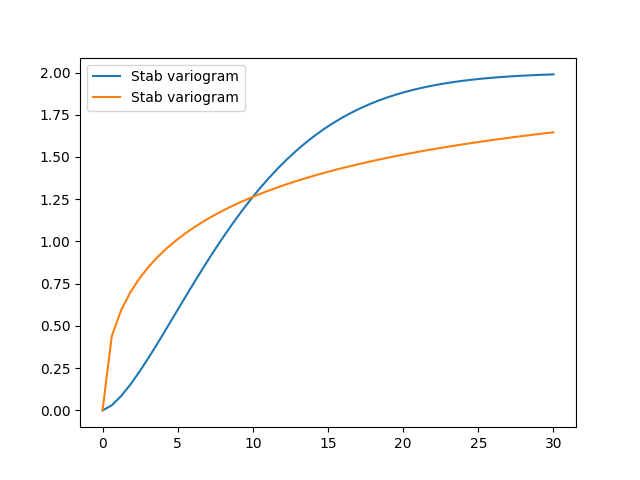Let’s pimp our self-defined model Gau from the introductory example by setting the exponent as an additional parameter:This leads to the so called stable covariance model and we can define it by

import numpy as np
import gstools as gs

class Stab(gs.CovModel):
def default_opt_arg(self):
return {"alpha": 1.5}

def cor(self, h):
return np.exp(-h ** self.alpha)


As you can see, we override the method CovModel.default_opt_arg to provide a standard value for the optional argument alpha. We can access it in the correlation function by self.alpha

Now we can instantiate this model by either setting alpha implicitly with the default value or explicitly:

model1 = Stab(dim=2, var=2.0, len_scale=10)
model2 = Stab(dim=2, var=2.0, len_scale=10, alpha=0.5)
ax = model1.plot()
model2.plot(ax=ax)Apparently, the parameter alpha controls the slope of the variogram and consequently the roughness of a generated random field.

Note

You don’t have to override the CovModel.default_opt_arg, but you will get a ValueError if you don’t set it on creation.

Total running time of the script: ( 0 minutes 0.140 seconds)

Gallery generated by Sphinx-Gallery